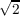#### Vol. 59, No. 1, 1975

 Download this articleFor screen For printingRecent Issues Vol. 325: 1 Vol. 324: 1  2 Vol. 323: 1  2 Vol. 322: 1  2 Vol. 321: 1  2 Vol. 320: 1  2 Vol. 319: 1  2 Vol. 318: 1  2Online Archive Volume: Issue:The Journal Subscriptions Editorial Board Officers Contacts Submission Guidelines Submission Form Policies for Authors ISSN: 1945-5844 (e-only) ISSN: 0030-8730 (print) Special Issues Author Index To Appear Other MSP Journals
On Gross differentiation on Banach spaces

### Hui-Hsiung Kuo

Vol. 59 (1975), No. 1, 135–145
##### Abstract

Let pt(x,) denote the Wiener measure in an abstract Wiener space (H,B) with variance parameter t > 0 and mean x in B. It is shown that if f L2pt(x,),t > 0 and x are fixed, then the function ptf defined by ptf(x + h) = Bf(y)pt(x + h,dy) for h in H is infinitely Gross differentiable at x. The first two derivatives are given by (Dptf(x),h) = t1 Bf(y)(h,y x)pt(x,dy) and (D2ptf(x)k,h) = t1 Bf(y){t1(h,y x)(k,y x) (h,k)}pt(x,dy), where h and k are in H. Moreover, D2ptf(x) is a Hilbert-Schmidt operator and D2ptf(x)2t1{ B|f(y)|2pt(x,dy)}12. An application to Uhlenbeck-Ornstein process is also given.

##### Mathematical Subject Classification 2000
Primary: 28A40
Secondary: 60J65, 58D15
##### Milestones